# Pandas DataFrame Plot line graph

Pandas plot

Pandas.DataFrame.plot to get line graphs using data

Python Pandas Plot Line graph by using DataFrame from Excel file with options & to save as image

Let us create a DataFrame with name of the students and their marks.
``````import pandas as pd
my_dict={
'NAME':['Ravi','Raju','Alex','Ron','Geek','Kim'],
'MARK':[20,30,40,30,40,50]
}
my_df = pd.DataFrame(data=my_dict)
print(my_df)``````
Output is here
``````   NAME  MARK
0  Ravi    20
1  Raju    30
2  Alex    40
3   Ron    30
4  Geek    40
5   Kim    50``````

## Line Graph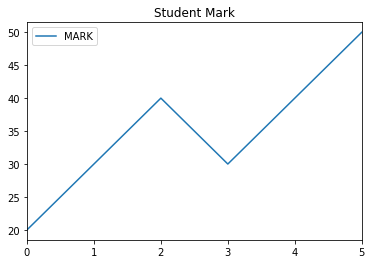``````import pandas as pd
my_dict={
'NAME':['Ravi','Raju','Alex',
'Ron','Geek','Kim'],
'MARK':[20,30,40,30,40,50]
}
df = pd.DataFrame(data=my_dict)
df.plot.line(title="Std Mark");``````

## Options

There are several options we can add to above plot. We will discuss them here. Before that let us try some of the options for our line graph.
``````import pandas as pd
my_dict={
'NAME':['Ravi','Raju','Alex',
'Ron','Geek','Kim'],
'MARK':[20,30,40,30,40,50]
}
df = pd.DataFrame(data=my_dict)
my_style=['-.']
df.plot.line( title="Student Mark",figsize=(6,3),x='NAME',y='MARK',
use_index=True,grid=True,legend=True,style=my_style)``````## title :

title='Student Mark' String used as Title of the graph. We can also use one list to give titles to sub graphs. ( for this subplot must be true )

## figsize :

Size of the graph , it is a tuple saying width and height in inches, figsize=(6,3). Here width is 6 inches and height is 3 inches.

## X: Y:

What is to be used in X and Y axis. If you have multiple keys then you can specify what can be used. Note that Y axis must be numeric data to plot the graph.
Example : Here we have used y='ENGLISH' to plot the graph against the name of students ( x='NAME' )
``````import pandas as pd
my_dict={
'NAME':['Ravi','Raju','Alex',
'Ron','Geek','Kim'],
'MARK':[20,30,40,30,40,50],
'ENGLISH':[80,70,60,70,90,80]
}
df = pd.DataFrame(data=my_dict)
df.plot.line( title="Student Mark",x='NAME',y='ENGLISH',use_index=True,legend=True)``````

## use_index

True or False ( default is True ) , To show index or not. The difference is mentioned here ( check X - axis ) .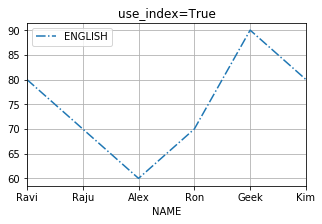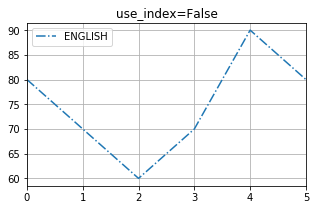## grid

We will show grid ( grid=True ) or not ( grid=False)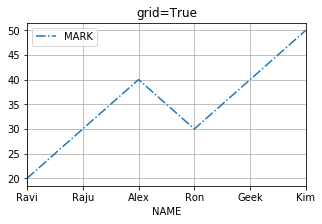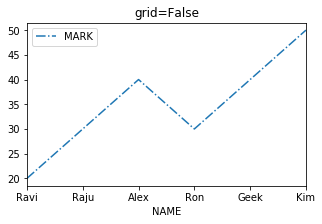## legend

True of False ( default is True ), to show legend ( legend=True ) or not ( legend=False)## style

This is a list or dict. We can specify how the style of the line.
``````my_style=[':']
df.plot.line( title="style =[ ':'] ",x='NAME',style=my_style)``````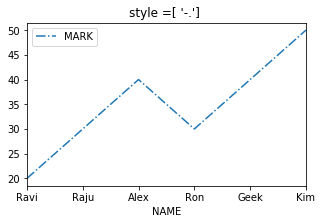## logx logy loglog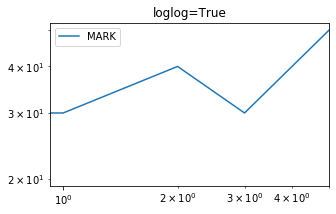We can specify log scaling or symlog scaling for x ( logx=True ) or y ( logy=True ) or for both x & y ( loglog=True)

``df.plot.line( title="loglog=True",figsize=(5,3),loglog=True)``

## secondary_y

Whether to plot on secondary Y Axis ( secondary_y=True ) or not ( secondary_y=False )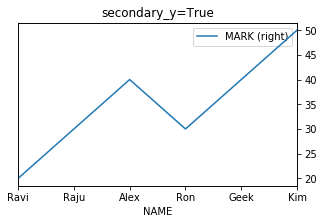## mark_right

Check the image above when secondary_y=True. There is a automatic marking in column lebels saying (right). We can manage this to show ( mark_right=True) or not ( mark_right=False)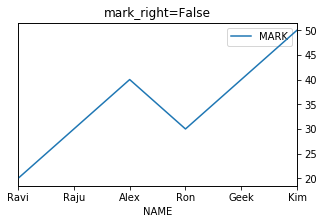``df.plot.line( title="secondary_y=True",x='NAME',figsize=(5,3),secondary_y=True)``

## rot

Rotation of ticks ( check the names at x axis )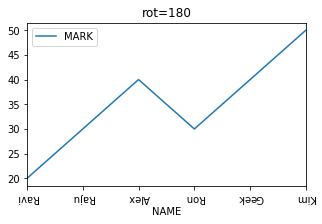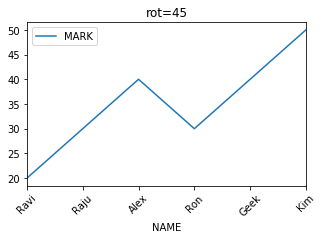``df.plot.line( title="rot=180",x='NAME',figsize=(5,3),rot=180)``

Subscribe to our YouTube Channel here

## Subscribe

* indicates required
Subscribe to plus2netplus2net.com